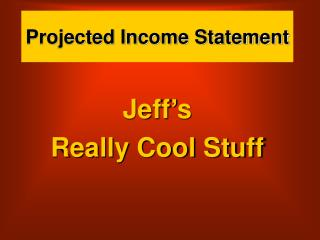DownloadDownload PresentationProjected Income Statement

# Projected Income Statement

Télécharger la présentation## Projected Income Statement

- - - - - - - - - - - - - - - - - - - - - - - - - - - E N D - - - - - - - - - - - - - - - - - - - - - - - - - - -
##### Presentation Transcript

1. Projected Income Statement Jeff’s Really Cool Stuff

2. Assumptions • Percentage of Gross Income before Tax to Revenue = 15% • My tax rate = 25% • Cost of Goods Sold = 40% • Inventory Turnover - 37

3. Assumptions • I want to have Net Income AFTER Tax of \$60,000

4. Calculations Net Income After Tax \$60,000 \$60,000 divided by the inverse (I minus the tax rate expressed as a decimal) of the tax rate = \$60,000 divided by 1 minus .25 = \$60,000 divided by .75 = Gross Income Before Tax of \$80,000 Gross Income Before Tax \$80,000 Tax (25%) \$20,000

5. Calculations Net Income After Tax \$60,000 Revenue \$? = 15% Gross Income Before Tax \$80,000 Tax (25%) \$20,000

6. Calculations Net Income After Tax \$60,000 Revenue \$ 533,334 \$80,000 divided by the Percentage of Net Income before Tax to Revenue = \$80,000 divided by .15 = \$533,334 Gross Income Before Tax \$80,000 Tax (25%) \$20,000

7. Calculations Net Income After Tax \$60,000 Revenue \$ 533,334 Cost of Goods Sold = 40%, so .4 times \$533,334 = \$213,334 Cost of Goods Sold \$213,334 Gross Income Before Tax \$80,000 Tax (25%) \$20,000

8. Calculations Net Income After Tax \$60,000 Revenue \$ 533,334 Cost of Goods Sold \$213,334 Gross Profit \$320,000 \$240,000 Operating Expenses \$? Gross Income Before Tax \$80,000 Tax (25%) \$20,000

9. Calculations Revenue \$ 533,334 Inventory Turnover ratio = 37 Inventory Turnover ratio = Cost of Goods Sold divided by Average Inventory (Beginning Inventory plus Ending Inventory) divided by 2 Cost of Goods Sold \$213,334

10. Calculations Revenue \$ 533,334 Beginning Inv \$5,766 \$213,334 divided by \$? = 37 \$213,334 divided by 37 = Ave Inven \$213,334 divided by 37 = \$5,766 Cost of Goods Sold \$213,334

11. Calculations Revenue \$ 533,334 Beginning Inv \$5,766 Purchases \$213,334 GAS \$219,100 Ending Inv \$5,766 Cost of Goods Sold \$213,334